Search Results
• #### Let the signals x(t) and y(t) be the input and output signals to a differentiator, respectively....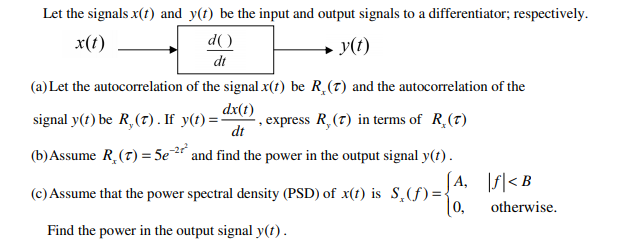Let the signals x(t) and y(t) be the input and output signals to a differentiator, respectively. x(t) do y(t) (a) Let the autocorrelation of the signal x(t) be R (T) and the autocorrelation of the signal y(t) be R (T). If y(t)= X, express R, (T) in terms of R. (T) dt (b) Assume R (T) = 5e and find...

• #### 151 4.8 Problems 4.17 The autocorrelation function Txx(t) of a real analog signal x (t) is defined by xx(t) x(t)x...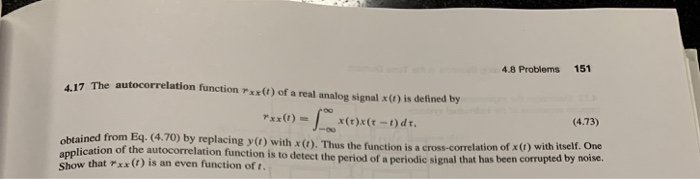151 4.8 Problems 4.17 The autocorrelation function Txx(t) of a real analog signal x (t) is defined by xx(t) x(t)x (r-t) dt, (4.73) obtained from Eq. (4.70) by replacing y(t) with x (t). Thus the function is a cross-correlation of x(t) with itself. One application of the autocorrelation function is to detect the period of a periodic signal that has...

• #### 1.12. The Fourier transform of a signal x(t) is defined by X(f) = sincf, where the sinc func- tion is as defined in Equ...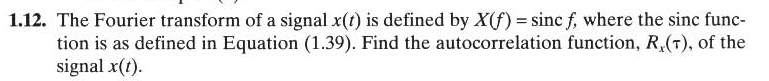1.12. The Fourier transform of a signal x(t) is defined by X(f) = sincf, where the sinc func- tion is as defined in Equation (1.39). Find the autocorrelation function, R.(T), of the signal x(t). 1.12. The Fourier transform of a signal x(t) is defined by X(f) = sincf, where the sinc func- tion is as defined in Equation (1.39). Find...

• #### Consider a sinusoidal signal with random phase, defined by x(t)=Acos(2πfct+θ), where A and FC are constant and θ is a random variable uniform distributed over interval [-π,π], that is f(θ)={█(1/2π,&-π≤θ≤π@0,&elsewhere)┤ Describe the autocorrelation RX(τ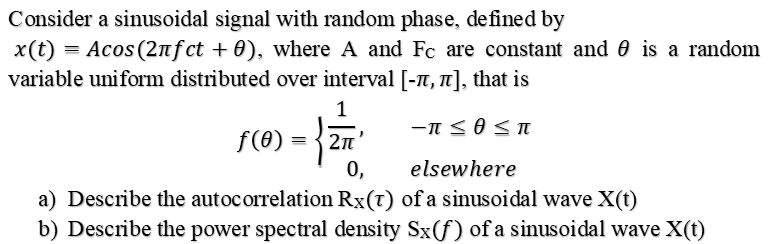Consider a sinusoidal signal with random phase, defined by , where A and FC are constant and  is a random variable uniform distributed over interval [-, that isa)    Describe the autocorrelation RX of a sinusoidal wave X(t)b)    Describe the power spectral density SX of a sinusoidal wave X(t)Consider a sinusoidal signal with random phase, defined by , where A and...

• #### Consider the RC circuit shown below. Assume that R=(0.1)2 and C=(0.1)F 3. R i(t) y (t)...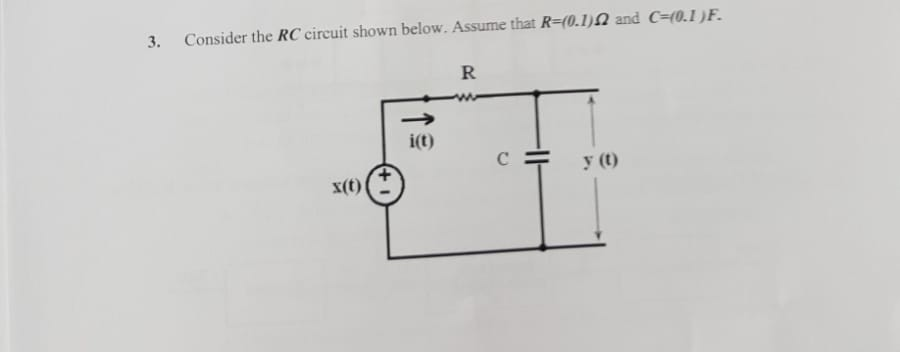Consider the RC circuit shown below. Assume that R=(0.1)2 and C=(0.1)F 3. R i(t) y (t) x(t) The input to this circuit is given as x(t) s(t)+ny (t), where the noise component of input, n(t), is a sample function realization of white noise process with an autocorrelation function given by Rpx(t) 8(T), and s (t) cos(6Tt) is the signal component...

• #### Consider the RC circuit shown below. Assume that R=(0.1)2 and C=(0.1)F 3. R i(t) y (t)...Consider the RC circuit shown below. Assume that R=(0.1)2 and C=(0.1)F 3. R i(t) y (t) x(t) The input to this circuit is given as x(t) s(t)+ny (t), where the noise component of input, n(t), is a sample function realization of white noise process with an autocorrelation function given by Rpx(t) 8(T), and s (t) cos(6Tt) is the signal component...

• #### 9. Random binary signal x(t) transmit one digit every Tb seconds. A binary bit '1, is transmitted...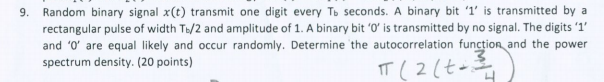9. Random binary signal x(t) transmit one digit every Tb seconds. A binary bit '1, is transmitted by a rectangular pulse of width T&/2 and amplitude of 1. A binary bit 'O' is transmitted by no signal. The digits1 and '0' are equal likely and occur randomly. Determine the autocorrelation function and the power spectrum density. (20 points) 3 9....

• #### 4. Find and the autocorrelation function R (t) of the following signal : x( Find the...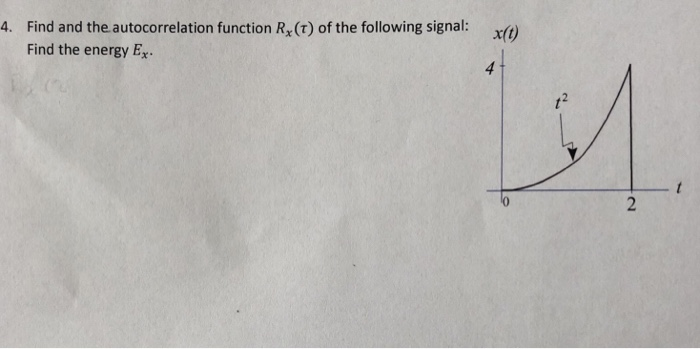4. Find and the autocorrelation function R (t) of the following signal : x( Find the energy Ex

• #### 1) Random Processes: Suppose that a wide-sense stationary Gaussian random process X (t) is input to the filter shown below. The autocorrelation function of X(t) is 2xx (r) = exp(-ary Y(t) X(t) Delay...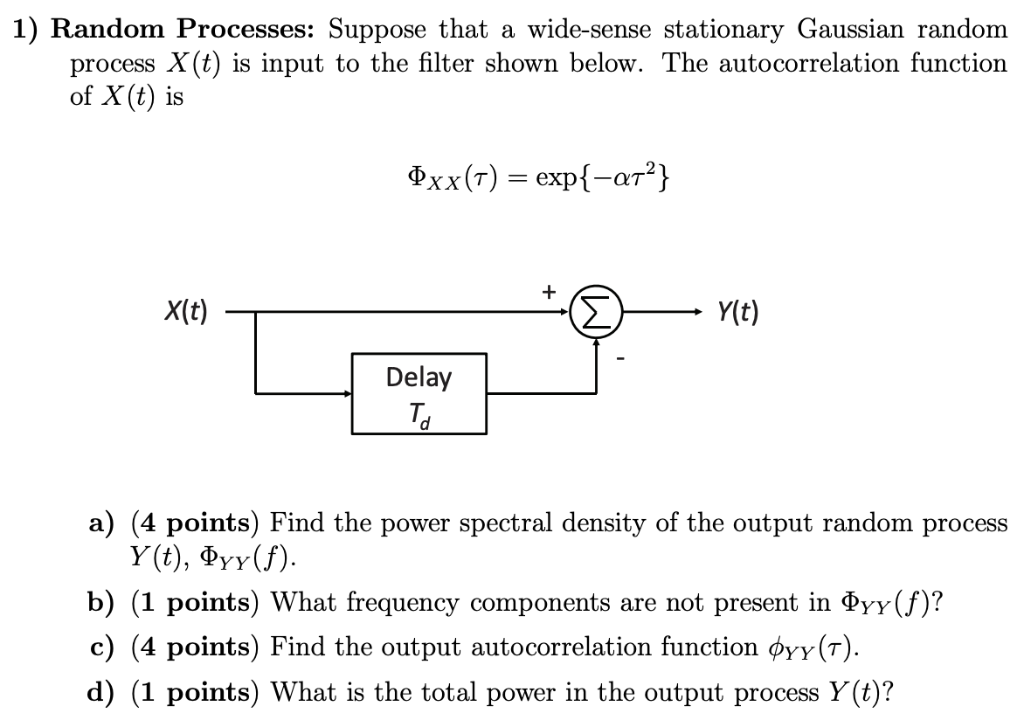1) Random Processes: Suppose that a wide-sense stationary Gaussian random process X (t) is input to the filter shown below. The autocorrelation function of X(t) is 2xx (r) = exp(-ary Y(t) X(t) Delay a) (4 points) Find the power spectral density of the output random process y(t), ΦΥΥ(f) b) (1 points) What frequency components are not present in ΦYYU)? c)...

• #### 3. A white Gaussian noise signal W (t) with autocorrelation function it passes through a linear filter invariant in time h (t). Calculate the average power of the exit process Y (t) knowing that We w...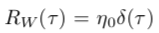3. A white Gaussian noise signal W (t) with autocorrelation function it passes through a linear filter invariant in time h (t). Calculate the average power of the exit process Y (t) knowing that We were unable to transcribe this imager. h2 (t)dt = 1. r. h2 (t)dt = 1.